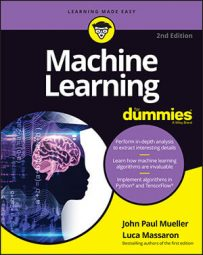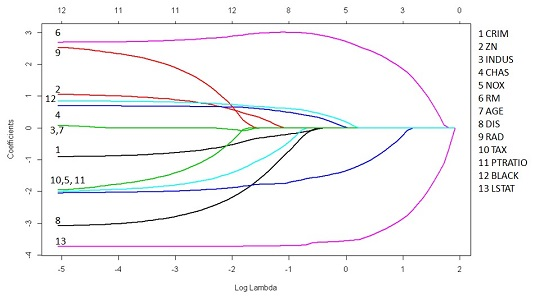##### Machine Learning For DummiesHaving many features to work with may seem to address the need for machine learning to understand a problem fully. However, just having features doesn’t solve anything; you need the right features to solve problems. Keep reading to ensure that you have the right features when performing machine learning tasks.

## Defining the outcome of features that don’t work together

As previously mentioned, having many features and making them work together may incorrectly indicate that your model is working well when it really isn’t. Unless you use cross-validation, error measures such as R2 can be misleading because the number of features can easily inflate it, even if the feature doesn’t contain relevant information. The following example shows what happens to R2 when you add just random features.

`from sklearn.cross_validation import train_test_split`

`from sklearn.metrics import r2_score`

`X_train, X_test, y_train, y_test = train_test_split(X,`

`y, test_size=0.33, random_state=42)`

`check = [2**i for i in range(8)]`

`for i in range(2**7+1):`

`X_train = np.column_stack((X_train,np.random.random(`

`X_train.shape)))`

`X_test = np.column_stack((X_test,np.random.random(`

`X_test.shape)))`

`regression.fit(X_train, y_train)`

`if i in check:`

`print ("Random features: %i -> R2: %0.3f" %`

`(i, r2_score(y_train,regression.predict(X_train))))`

What seems like an increased predictive capability is really just an illusion. You can reveal what happened by checking the test set and discovering that the model performance has decreased.

`regression.fit(X_train, y_train)`

`print ('R2 %0.3f'`

`% r2_score(y_test,regression.predict(X_test)))`

`# Please notice that the R2 result may change from run to`

`# run due to the random nature of the experiment`

` `

`R2 0.474`

## Solving overfitting by using selection

Regularization is an effective, fast, and easy solution to implement when you have many features and want to reduce the variance of the estimates due to multicollinearity between your predictors. Regularization works by adding a penalty to the cost function. The penalization is a summation of the coefficients. If the coefficients are squared (so that positive and negative values can’t cancel each other), it’s an L2 regularization (also called the Ridge). When you use the coefficient absolute value, it’s an L1 regularization (also called the Lasso).

However, regularization doesn’t always work perfectly. L2 regularization keeps all the features in the model and balances the contribution of each of them. In an L2 solution, if two variables correlate well, each one contributes equally to the solution for a portion, whereas without regularization, their shared contribution would have been unequally distributed.

Alternatively, L1 brings highly correlated features out of the model by making their coefficient zero, thus proposing a real selection among features. In fact, setting the coefficient to zero is just like excluding the feature from the model. When multicollinearity is high, the choice of which predictor to set to zero becomes a bit random, and you can get various solutions characterized by differently excluded features. Such solution instability may prove a nuisance, making the L1 solution less than ideal.

Scholars have found a fix by creating various solutions based on L1 regularization and then looking at how the coefficients behave across solutions. In this case, the algorithm picks only the stable coefficients (the ones that are seldom set to zero). You can read more about this technique on the Scikit-learn website. The following example modifies the polynomial expansions example using L2 regularization (Ridge regression) and reduces the influence of redundant coefficients created by the expansion procedure:

from sklearn.preprocessing import PolynomialFeatures

`from sklearn.cross_validation import train_test_split`

` `

`pf = PolynomialFeatures(degree=2)`

`poly_X = pf.fit_transform(X)`

`X_train, X_test, y_train, y_test =`

`train_test_split(poly_X,`

`y, test_size=0.33, random_state=42)`

` `

`from sklearn.linear_model import Ridge`

`reg_regression = Ridge(alpha=0.1, normalize=True)`

`reg_regression.fit(X_train,y_train)`

`print ('R2: %0.3f'`

`% r2_score(y_test,reg_regression.predict(X_test)))`

` `

`R2: 0.819`

The next example uses L1 regularization. In this case, the example relies on R because it provides an effective library for penalized regression called glmnet. You can install the required support using the following command:

`install.packages("glmnet")`

Stanford professors and scholars Friedman, Hastie, Tibshirani, and Simon created this package. Prof. Trevor Hastie actually maintains the R package. The example shown below tries to visualize the coefficient path, representing how the coefficient value changes according to regularization strength. The lambda parameter decides the regularization strength. As before, the following R example relies on the Boston dataset, which is obtained from the MASS package.

`data(Boston, package="MASS")`

`library(glmnet)`

`X <- as.matrix(scale(Boston[,1:ncol(Boston)-1]))`

`y <- as.numeric(Boston[,ncol(Boston)])`

`fit = glmnet(X, y, family="gaussian", alpha=1,`

`standardize=FALSE)`

`plot(fit, xvar="lambda", label=TRUE, lwd=2)`Visualizing the path of coefficients using various degrees of L1 regularization.

The chart represents all the coefficients by placing their standardized values on the ordinate, the vertical axis. As for as the abscissa, the scale uses a log function of lambda so that you get an idea of the small value of lambda at the leftmost side of the chart (where it’s just like a standard regression). The abscissa also shows another scale, placed in the uppermost part of the chart, that tells you how many coefficients are nonzero at that lambda value.

Running from left to right, you can observe how coefficients decrease in absolute value until they become zero, telling you the story of how regularization affects a model. Naturally, if you just need to estimate a model and the coefficients for the best predictions, you need to find the correct lambda value using cross-validation:

`cv <- cv.glmnet(X, y, family="gaussian",`

`alpha=1, standardize=FALSE)`

`coef(cv, s="lambda.min")`